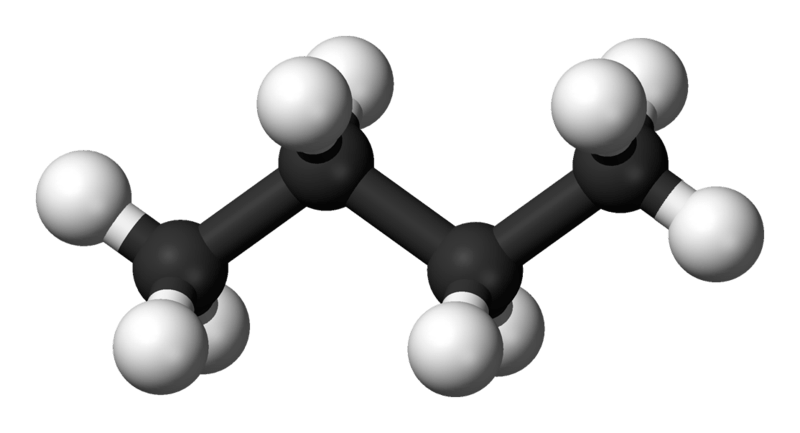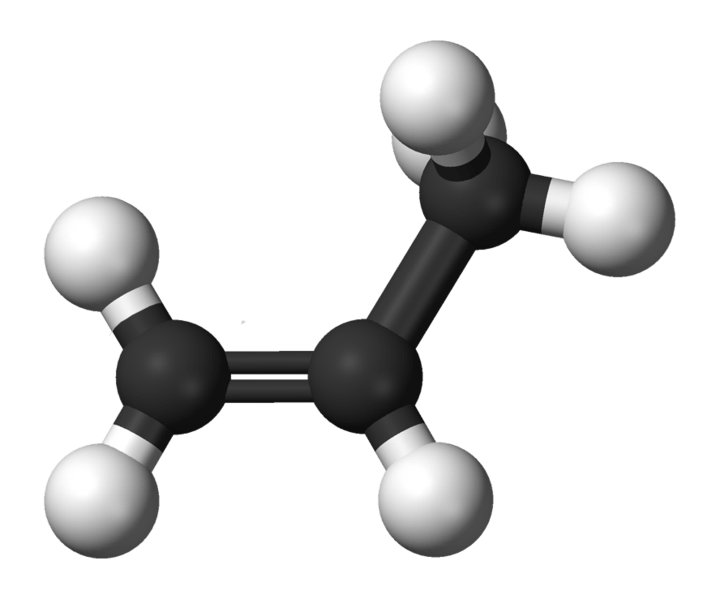# Difference Between Formula Mass and Molecular Mass

## Main Difference – Formula Mass vs Molecular Mass

Formula mass and molecular mass are two terms used to find the mass of a molecule. The formula mass gives the mass of a molecule when the empirical formula of that molecule is considered. The molecular mass gives the mass of a molecule when the molecular formula of that molecule is considered. In both methods, the mass is calculated by adding the masses of each atom present in that formula. The main difference between formula mass and the molecular mass is that formula mass is calculated by adding the masses of atoms present in the simplest formula that can be given for a molecule whereas molecular mass is calculated using the actual numbers of atoms present in a molecule.

### Key Areas Covered

1. What is Formula Mass
– Definition, Calculations with Examples
2. What is Molecular Mass
– Definition, Calculations with Examples
3. What is the Difference Between Formula Mass and Molecular Mass
– Comparison of Key Differences

Key Terms: Amu, Empirical Formula, Formula Mass, Mole, Molar Mass, Molecular Mass## What is Formula Mass

Formula mass is the mass of a molecule calculated using the empirical formula of that molecule. The empirical formula is the formula that shows all types of atoms (elements) present in a molecule and their ratios. This means it represents the simplest formula that can be given for a molecule. Sometimes, the empirical formula and the molecular formula are the same for small molecules.

### Calculation of Formula mass

Formula mass is the sum of the masses of the atoms present in the empirical formula. It is calculated using the unit “amu” (atomic mass units). Each and every atom has a mass given by amu units. 1 amu is equal to 1.66 x 10-24 g. Let us consider few examples in order to understand how to calculate the formula mass.

Ex: The formula mass for MgCl2.

The empirical formula for MgCl2 is the same as the molecular formula. Therefore, the formula mass can be calculated as follows.

The mass of Mg atom            = 24.305 amu
The mass of a Cl atom           = 35.453 amu
Therefore the formula mass = (24.305 amu) + (2 x 35.453 amu)
= 95.211 amu

Ex: The formula mass of C4H10

The empirical formula for C4H10 is C2H5. Therefore, the formula mass can be calculated as follows.

The mass of C atom                 = 12.0107amu
The mass of an H atom             = 1.0079 amu
Therefore the formula mass  = (2 x 12.0107amu) + (5 x 1.0079 amu)
= 29.0609 amuFigure 1: C4H10

Likewise, the value of the formula mass is sometimes equal to the molecular mass, but sometimes it is less than molecular mass. But the formula mass cannot be higher than the molecular mass.

## What is Molecular Mass

The molecular mass of a molecule is the mass of a mole of that molecule. This means it is the sum of the masses of molecules present in a mole. This is calculated using the molecular formula of a molecule. Here, the atomic weights of each atom in g/mol unit are added to get the molecular mass.

One mole of a molecule is composed of 6.023 x 1023 molecules. Therefore, the molecular mass is the weight of 6.023 x 1023 molecules. Since the atomic masses of each element are known, it is easy to calculate the molecular mass rather than thinking of a 6.023 x 1023 of molecules.

### Calculation of Molecular Mass

Let us consider some examples.

Ex: Molecular mass of HCl

The atomic weight of H         = 1.0079 g/mol
The atomic weight of Cl        = 35.453 g/mol
The molecular mass of HCl  = (1.0079 g/mol) + (35.453 g/mol)
= 36.4609 g/mol.

Ex: Molecular mass of C3H6

The atomic weight of C             = 12.0107 g/mol
The atomic weight of H            = 1.0079 g/mol
The molecular mass of C3H6  = (3 x 12.0107 g/mol) + (6 x 1.0079 g/mol)
= 42.0795 g/molFigure 2: C3H6

The molecular mass of a molecule is also called the molar mass. This is because the mass is given per one mole.

## Difference Between Formula Mass and Molecular Mass

### Definition

Formula Mass: Formula mass is the sum of the masses of atoms present in the empirical formula.

Molecular Mass: Molecular mass of a molecule is the mass of a mole of that molecule.

### Units

Formula Mass: The formula mass is calculated from amu units.

Molecular Mass: The molecular mass is calculated from g/mol units.

### Calculation

Formula Mass: The formula mass is calculated using the empirical formula.

Molecular Mass: The molecular mass is calculated using the molecular formula.

### Value

Formula Mass: The formula mass may or may not give the exact mass of a molecule.

Molecular Mass: The molecular mass always give the exact mass of a mole of molecules.

### Conclusion

Formula mass and molecular mass of a molecule can easily be calculated using the atomic masses of the atoms that are present in the molecule. But the formula mass is calculated using the empirical formula whereas the molecular mass is calculated using the actual formula. Sometimes the empirical formula and the molecular formula are the same for a molecule. Then, the formula mass and molecular mass are the same. The main difference between formula mass and the molecular mass is that the formula mass is calculated by adding the masses of atoms present in the simplest formula that can be given for a molecule whereas the molecular mass is calculated using the actual numbers of atoms present in a molecule.

##### References:

1. Helmenstine, Ph.D. Anne Marie. “Review This Sample Problem to Find Molecular Mass of a Compound.” ThoughtCo, Available here. Accessed 13 Sept. 2017.
2. Helmenstine, Ph.D. Anne Marie. “What is the Difference between Formula Mass and Molecular Mass?” ThoughtCo, Available here. Accessed 13 Sept. 2017.
3. “Molar mass.” Wikipedia, Wikimedia Foundation, 23 Aug. 2017, Available here. Accessed 13 Sept. 2017.

##### Image Courtesy:

1. “Butane-3D-balls” By Ben Mills and Jynto – Derivative of File:Propan-1-ol-3D-balls.png. (Public Domain) via Commons Wikimedia
2. “Propylene-3D-balls” By Ben Mills and Jynto – Derivative of File:Cis-but-2-ene-3D-balls.png. (Public Domain) via Commons Wikimedia